Symmetric algebra

(diff) ← Older revision | Latest revision (diff) | Newer revision → (diff)

A generalization of a polynomial algebra. Ifis a unital module (cf. Unitary module) over a commutative associative ringwith an identity, then the symmetric algebra ofis the algebra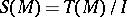, whereis the tensor algebra ofandis the ideal generated by the elements of the form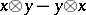(). A symmetric algebra is a commutative associative-algebra with an identity. It is graded: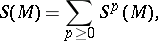where, and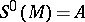,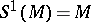. The module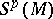is called the-th symmetric power of the module. Ifis a free module with finite basis, then the correspondence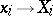() extends to an isomorphism of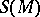onto the polynomial algebra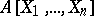(see Ring of polynomials).

For any homomorphism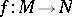of-modules, the-th tensor power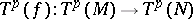induces a homomorphism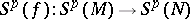(the-th symmetric power of the homomorphism). A homomorphism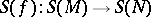of-algebras is obtained. The correspondencesand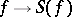are, respectively, covariant functors from the category of-modules into itself and into the category of-algebras. For any two-modulesandthere is a natural isomorphism.

Ifis a vector space over a field of characteristic 0, then the symmetrization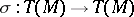(cf. Symmetrization (of tensors)) defines an isomorphism from the symmetric algebraonto the algebra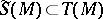of symmetric contravariant tensors overrelative to symmetric multiplication:How to Cite This Entry:
Symmetric algebra. Encyclopedia of Mathematics. URL: http://encyclopediaofmath.org/index.php?title=Symmetric_algebra&oldid=18728
This article was adapted from an original article by A.L. Onishchik (originator), which appeared in Encyclopedia of Mathematics - ISBN 1402006098. See original article